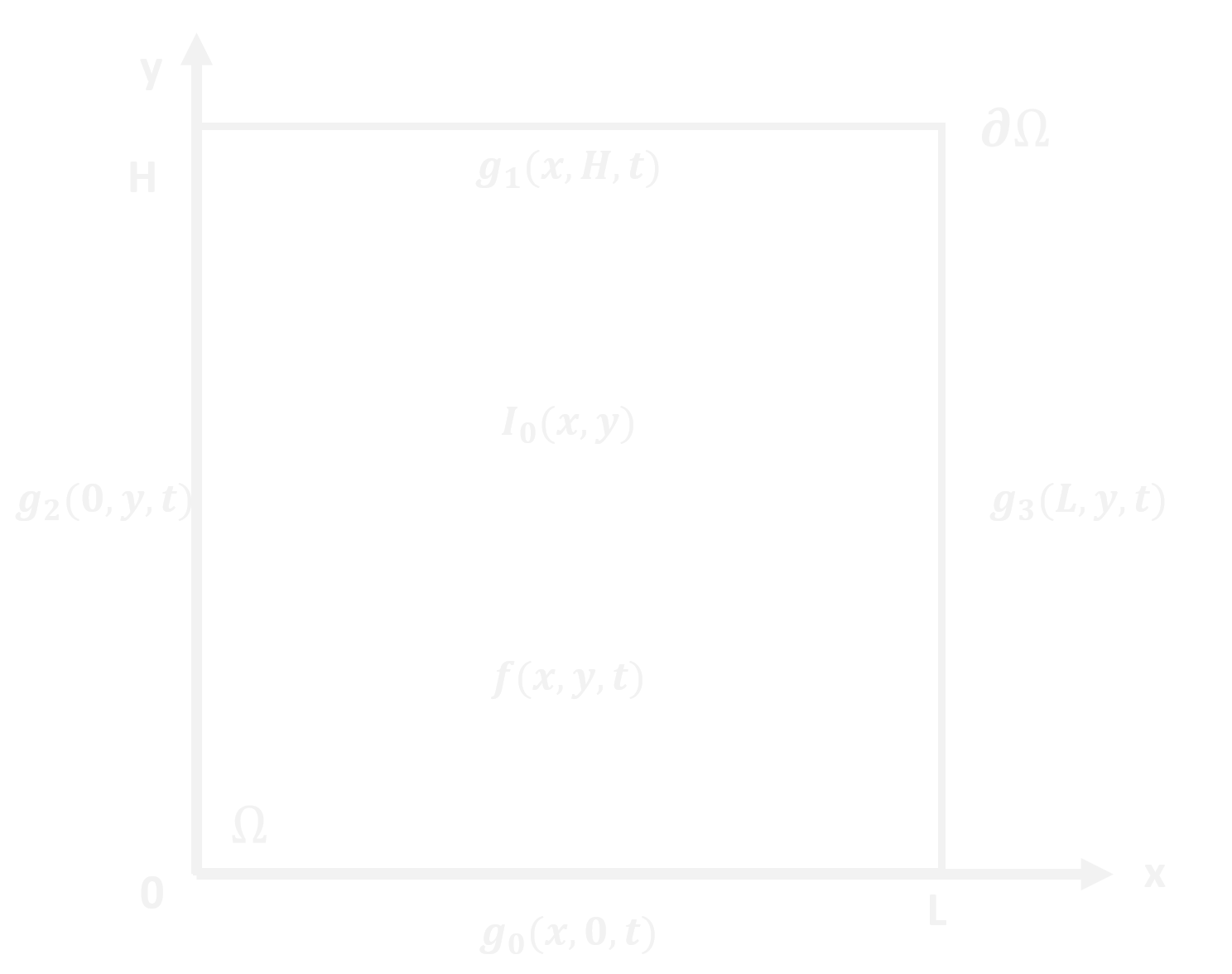#Diffusion2D_Library 2.0.0

.NET 5.0
`dotnet add package Diffusion2D_Library --version 2.0.0`
`NuGet\Install-Package Diffusion2D_Library -Version 2.0.0`
This command is intended to be used within the Package Manager Console in Visual Studio, as it uses the NuGet module's version of Install-Package.
`<PackageReference Include="Diffusion2D_Library" Version="2.0.0" />`
For projects that support PackageReference, copy this XML node into the project file to reference the package.
`paket add Diffusion2D_Library --version 2.0.0`
`#r "nuget: Diffusion2D_Library, 2.0.0"`
#r directive can be used in F# Interactive and Polyglot Notebooks. Copy this into the interactive tool or source code of the script to reference the package.
```// Install Diffusion2D_Library as a Cake Addin

// Install Diffusion2D_Library as a Cake Tool
#tool nuget:?package=Diffusion2D_Library&version=2.0.0```

## Diffusion2D_Library

Solves the diffusion equation in 1 or 2 dimensions.

"Diffusion2D_Library" is a C# library for solving parabolic partial differential equations in 1 or 2 dimensions. A representative region, Ω, in the cartesian plane, with boundary conditions specified on δΩ and initial condition indicated, is shown to the right.

The classic examples for parabolic partial differential equations are the heat and the diffusion equations. The solutions are subject to an initial and boundary conditions. The differences lie in the physical interpretation of the terms that make up the ν parameter and the types of boundary conditions imposed. We will focus on solutions to the diffusion equation in this write-up.

### Numerical Solvers

The region, Ω, is discretized in space such that Δx = Δy and there are Nx grid points in the x-direction and Ny grid points in the y-direction. Time is discretized into time-steps of Δt duration. Diffusion is calculated for Nt time-steps.

### Installation

The Diffusion2D_Library package can be obtained by using the nuget package manager in Visual Studio or by going to the NuGet link and using the provided commands.

### How to use the library

Once the library package has been added to a project, it can be invoked using the following code:

``````public void Test_2D_DiffusionSimulation()
{
string base_name = "Base_Output_Name"; //the iteration number will be appended to the name

double L = 100.0e-4;                // cm
double H = 100.0e-4;                // cm
int NX = 50;                        // Node number
int NY = 50;                        // Node number
double dx = L / NX;                 // cm
double dy = H / NY;                 // cm
double dt = 1.0e-4;                 // s
double Duration = 1.0e3;            // s
int NT = (int)(Duration / dt) + 1;  // Number of iterations
int Output = (int)(5.0 / dt);       // delay between writing output files, s
Mode VMode = Mode.verbose;          // Specifies whether output text is shown on the console

// Order of boundaries: Top, Right, Left, Bottom
ABoundaryCondition[] BCs = new ABoundaryCondition { ABoundaryCondition.dirichlet, ABoundaryCondition.neumann, ABoundaryCondition.neumann, ABoundaryCondition.neumann };
Del_BC_xy[] All_BCs = new Del_BC_xy { Boundary_2D_Constant, Boundary_2D_Zero, Boundary_2D_Zero, Boundary_2D_Zero };

Del_IC_xy IC2 = InitialCondition_2D_Zero;

Del_Source_MatrixD f = SourceTerm_2D_Zero;

// Specify the diffusion coefficient at each node
RMatrix D = new(NX, NY);
for (int i = 0; i < NX; i++)
{
for (int j = 0; j < NY; j++)
{
D[i, j] = 1.0e-8;          // cm2/sec
}
}
// Create a simulator for 2D Diffusion
DiffusionSimulators_2D_MatrixD diffusionSimulator_2D = new(D, dx, dx, NX, NY, dt, NT, BCs, All_BCs, IC2, f, VMode, base_name);

// Solve the diffusion equation for the given initial and boundary conditions
diffusionSimulator_2D.Solve(NT, Output);
// Note that the final composition field is stored as a property of the diffusion simulator class.  Intermediate composition fields were output to files during the simulation
}
``````

The source term function, initial condition function, and boundary condition functions are specified in the following manner:

#### Source Term

``````        public static RMatrix SourceTerm_2D_Zero(RMatrix xposition, RMatrix yposition, double time, RMatrix D, RMatrix composition)
{
int nrows = xposition.GetnRows;
int ncols = xposition.GetnCols;

RMatrix C_field = new(nrows, ncols);
return C_field;
}
``````

#### Initial Condiiton

``````        public static RMatrix InitialCondition_2D_Zero(RMatrix xposition, RMatrix yposition)
{
int nrows = xposition.GetnRows;
int ncols = xposition.GetnCols;
RMatrix composition_field = new(nrows, ncols);
return composition_field;
}
``````

#### Boundary Conditions

1. Example of a constant function
``````        public static RVector Boundary_2D_Constant(double t, RVector SideNodes, double XorYPositionValue)
{
int n = SideNodes.GetRVectorSize;
RVector BC = new(n);
for (int i = 0; i < n; i++)
{
BC[i] = 1.0;
}
return BC;
}
``````
1. Example of a zero-valued function
``````        public static RVector Boundary_2D_Zero(double t, RVector SideNodes, double XorYPositionValue)
{
int n = SideNodes.GetRVectorSize;
RVector BC = new(n);
for (int i = 0; i < n; i++)
{
BC[i] = 0.0;
}
return BC;
}
``````
Product Compatible and additional computed target framework versions.
.NET net5.0 is compatible.  net5.0-windows was computed.  net6.0 was computed.  net6.0-android was computed.  net6.0-ios was computed.  net6.0-maccatalyst was computed.  net6.0-macos was computed.  net6.0-tvos was computed.  net6.0-windows was computed.  net7.0 was computed.  net7.0-android was computed.  net7.0-ios was computed.  net7.0-maccatalyst was computed.  net7.0-macos was computed.  net7.0-tvos was computed.  net7.0-windows was computed.
Compatible target framework(s)
• #### net5.0

• No dependencies.

### NuGet packages

This package is not used by any NuGet packages.

### GitHub repositories

This package is not used by any popular GitHub repositories.